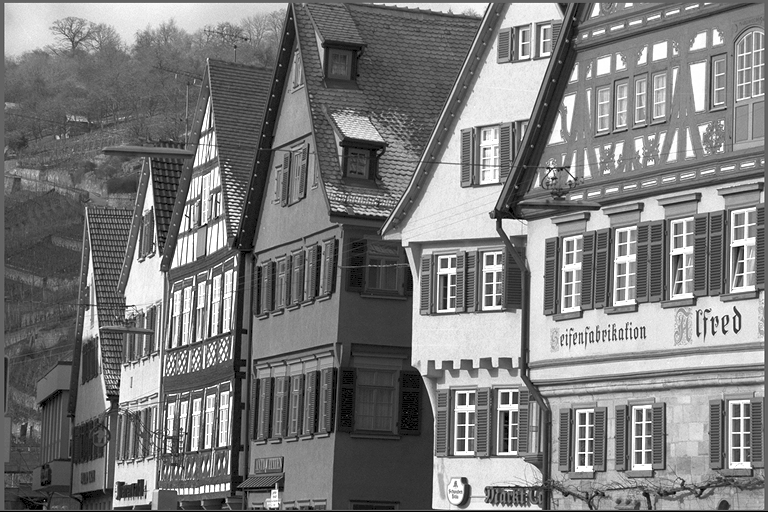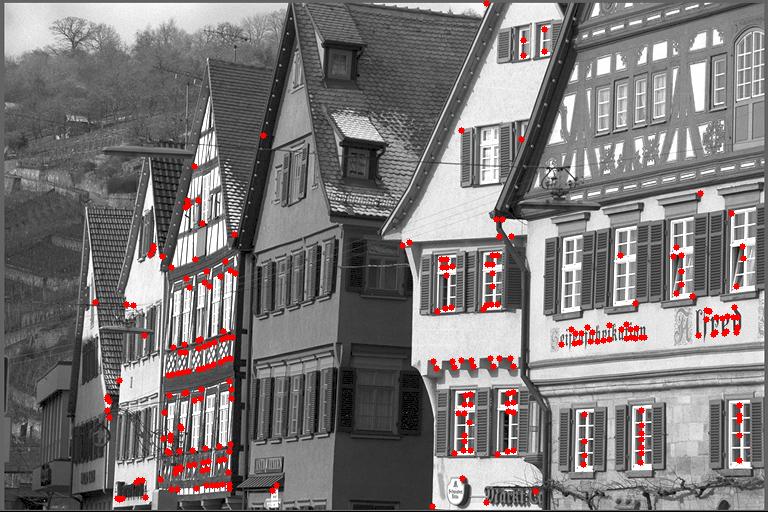## VPI - Vision Programming Interface

#### 2.2 Release

FAST Corners Detector

# Overview

Features from Accelerated Segment Test (FAST) is a corner detection algorithm. It detects corners on an input image, returning their coordinates. These corners can then be used as feature keypoints for tracking. The main advantage of using FAST is its computational efficiency, thus its name. This advantage is important in real-time video processing and machine learning pipelines. The main disadvantage of FAST is its lack of orientation and sensitivity to image domain and feature scale. The following table shows an example input image, a set of input parameters for the FAST algorithm (explained below), and the detected corners in red.

Input Parameters Output\begin{align*} \mathit{circleRadius} &= 3 \\ \mathit{arcLength} &= 9 \\ \mathit{intensityThreshold} &= 142 \\ \mathit{nonMaxSuppression} &= 1 \end{align*}# Implementation

The FAST algorithm does a corner detection test on all pixels of an input image. The algorithm was first described in  and later improved on . A pixel is detected to be a corner if a number of consecutive pixels around it are either brighter or darker than it.

The FAST algorithm defines pixels around a central, candidate pixel $$p$$ as those lying on a Bresenham circle $$x \in \{1...M\}$$ with radius $$R$$, produced by the function $$B(R, p)$$. The figure below shows an example of a candidate pixel (in yellow) and its Bresenham circle (in orange) with $$R = 3$$, and thus containing $$M = 16$$ pixels. The circle radius $$R$$ is a parameter of the algorithm (VPIFASTCornerDetectorParams::circleRadius) and it uniquely defines the $$M$$ pixels around the central pixel.

The intensity of the central pixel is denoted by $$I_p$$, and the intensity of pixels on the circle is denoted by $$I_{p\rightarrow x}$$. Each pixel on the circle can have one of three states: $$\text{brighter}$$; $$\text{darker}$$; or $$\text{similar}$$. The state of pixels on the circle is denoted by $$S_{p\rightarrow x}$$. The FAST algorithm tests if at least $$N$$ consecutive pixels in the circle, called an arc, have the same state of either $$\text{brighter}$$ or $$\text{darker}$$ than the candidate pixel, plus or minus a threshold $$t$$, respectively. The following equations summarize these concepts:

\begin{align*} S_{p\rightarrow x} &= \begin{cases} \text{brighter} &I_p + t \geq &I_{p\rightarrow x} & \\ \text{darker} & &I_{p\rightarrow x} \leq &I_p - t \\ \text{similar} &I_p - t < &I_{p\rightarrow x} < &I_p + t \\ \end{cases} \\ I_{p\rightarrow x} &= B(R, p) \end{align*}

The figure below shows an example of a candidate pixel (in yellow) and an arc in the circle around it (in red) with $$N = 9$$. The arc length $$N$$ and the intensity threshold $$t$$ are parameters of the algorithm (respectively: VPIFASTCornerDetectorParams::arcLength and VPIFASTCornerDetectorParams::intensityThreshold).

The FAST algorithm also provides a secondary test to remove neighbor corners called non-maximum suppression. If the non-maximum suppression parameter flag (VPIFASTCornerDetectorParams::nonMaxSuppression) is turned on, each pixel detected as a corner checks its immediate 1-rind neighborhood for another pixel also detected as a corner. If 2 or more neighbor pixels are marked as a corner, each is assigned a score value $$V$$ as the maximum threshold value that makes it remain a corner. The pixel with the maximum score $$V$$ survives the test, i.e. remains a corner, while the others are no longer corners. This effectively reduces the key-point corners found. This secondary test reduces the performance of the FAST algorithm.

# C API functions

For list of limitations, constraints and backends that implements the algorithm, consult reference documentation of the following functions:

Function Description
vpiInitFASTCornerDetectorParams Initializes VPIFASTCornerDetectorParams with default values.
vpiSubmitFASTCornerDetector Submits a FAST Corner Detector operation to the stream.

# Usage

Language:
1. Import VPI module
import vpi
2. Run FAST corner detector algorithm on the input image using the CPU backend.
with vpi.Backend.CPU:
corners = input.fastcorners()
3. Optionally, retrieve the first corner position found.
1. Lock the output corners array to efficiently access their contents.
with corners.rlock_cpu() as corners_data:
2. Retrieve the coordinates of the first corner location. The (x, y) coordinates are being swapped to (y, x) and converted into a tuple. This is equivalent to the (i, j) position in a matrix, suitable for 2D numpy array indexing.
corners_loc = tuple(corners_data.astype(int)[::-1])
1. Initialization phase
1. Include the header that defines the FAST algorithm functions and parameter structure.
Declares functions that implement support for FAST Corners.
2. Define the input image object.
VPIImage input = /*...*/;
struct VPIImageImpl * VPIImage
A handle to an image.
Definition: Types.h:256
3. Create the output array that will store the keypoints with the FAST corners. The output array capacity controls the maximum corners to be found. In this example, the output array capacity is set to $$1000$$.
VPIArray corners;
VPIStatus vpiArrayCreate(int32_t capacity, VPIArrayType type, uint64_t flags, VPIArray *array)
Create an empty array instance.
struct VPIArrayImpl * VPIArray
A handle to an array.
Definition: Types.h:232
@ VPI_ARRAY_TYPE_KEYPOINT_F32
VPIKeypointF32 element.
Definition: ArrayType.h:77
4. Create the stream where the algorithm will be submitted for execution.
VPIStream stream;
vpiStreamCreate(0, &stream);
struct VPIStreamImpl * VPIStream
A handle to a stream.
Definition: Types.h:250
VPIStatus vpiStreamCreate(uint64_t flags, VPIStream *stream)
Create a stream instance.
2. Processing phase
1. Initialize the parameter structure with user-provided parameters. In this example, the parameters are set to reflect the output above, that is $$R = 3, N = 9, t = 142, NMS = 1$$.
params.arcLength = 9;
params.intensityThreshold = 142;
params.nonMaxSuppression = 1;
float intensityThreshold
Threshold to select a pixel as being part of the arc in circle around a keypoint candidate.
Definition: FASTCorners.h:112
int32_t arcLength
Arc length in pixels over the circle to check central pixel is a corner.
Definition: FASTCorners.h:106
int8_t nonMaxSuppression
Whether to apply non-maximum suppression to remove corners too close together.
Definition: FASTCorners.h:120
Circle radius around a pixel to check if it is a corner.
Definition: FASTCorners.h:92
VPIStatus vpiInitFASTCornerDetectorParams(VPIFASTCornerDetectorParams *params)
Initializes VPIFASTCornerDetectorParams with default values.
Structure that defines the parameters for vpiSubmitFASTCornerDetector.
Definition: FASTCorners.h:82
2. Submit the algorithm and its parameters to the stream. It'll be executed by the CPU backend. In this example, the border limited is used to ignore pixels near image boundary.
VPIStatus vpiSubmitFASTCornerDetector(VPIStream stream, uint64_t backend, VPIImage input, VPIArray outCorners, const VPIFASTCornerDetectorParams *params, VPIBorderExtension border)
Submits a FAST Corner Detector operation to the stream.
@ VPI_BACKEND_CPU
CPU backend.
Definition: Types.h:92
@ VPI_BORDER_LIMITED
Consider image as limited to not access outside pixels.
Definition: Types.h:282
3. Optionally, wait until the processing is done.
vpiStreamSync(stream);
VPIStatus vpiStreamSync(VPIStream stream)
Blocks the calling thread until all submitted commands in this stream queue are done (queue is empty)...
3. Cleanup phase
1. Free resources held by the stream, the input image and the output array.
vpiArrayDestroy(corners);
void vpiArrayDestroy(VPIArray array)
Destroy an array instance.
void vpiImageDestroy(VPIImage img)
Destroy an image instance.
void vpiStreamDestroy(VPIStream stream)
Destroy a stream instance and deallocate all HW resources.

For more information, see FAST Corners in the "C API Reference" section of VPI - Vision Programming Interface.

# Performance

Performance benchmarks will be added at a later time.

# References

1. E. Rosten, T. Drummond (2006), "Machine learning for high speed corner detection"
in 9th European Conference on Computer Vision, vol. 1, pp. 430-443
2. E. Rosten, R. Porter, T. Drummond (2010), "Faster and better: a machine learning approach to corner detection"
in IEEE Transactions on Pattern Analysis and Machine Intelligence, vol. 32, pp. 105-119